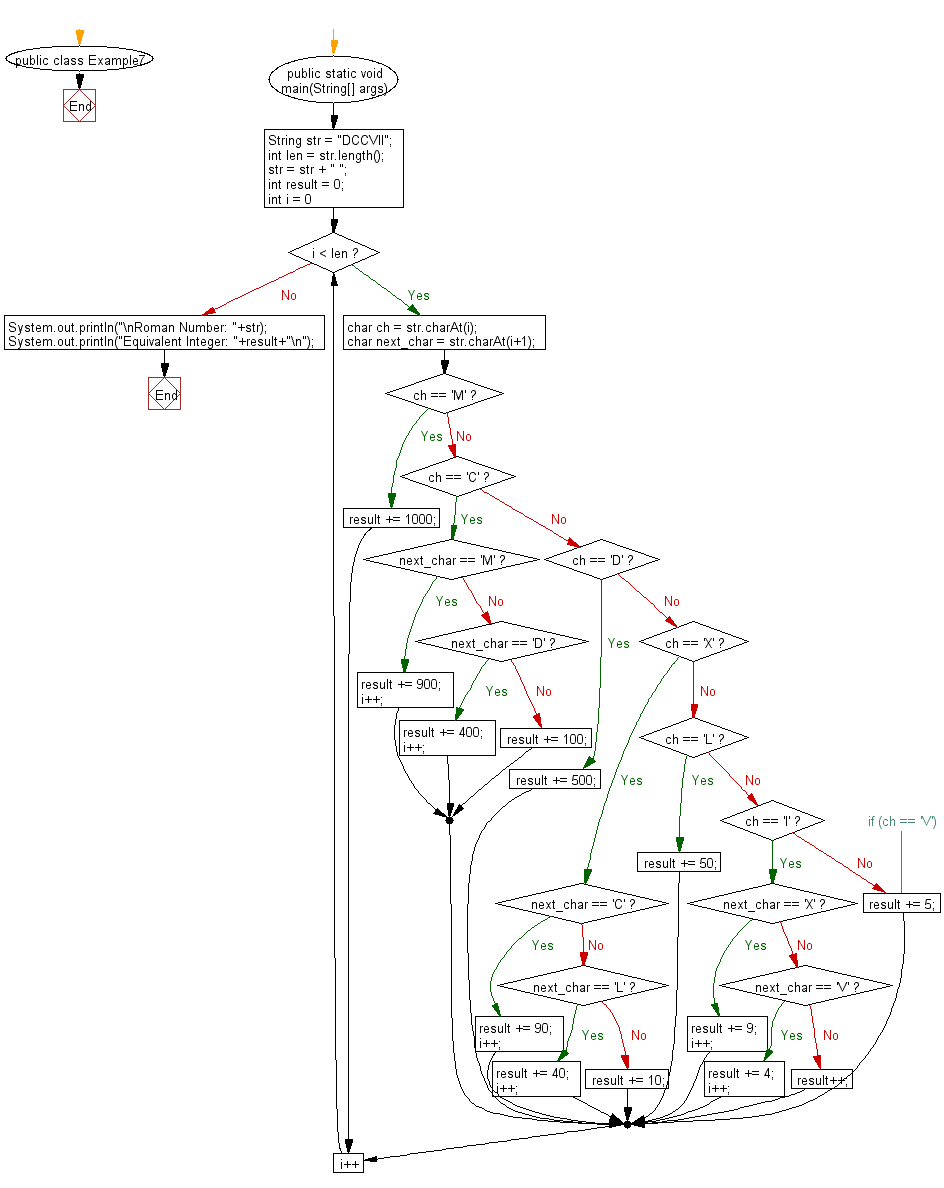﻿ Java Math Exercises: Convert Roman number to an integer number - w3resource# Java Math Exercises: Convert Roman number to an integer number

## Java Math Exercises: Exercise-7 with Solution

Write a Java program to convert Roman number to an integer number.

Sample Solution:

Java Code:

``````public class Example7 {
public static void main(String[] args) {
String str = "DCCVII";
int len = str.length();

str = str + " ";
int result = 0;
for (int i = 0; i < len; i++) {
char ch   = str.charAt(i);
char next_char = str.charAt(i+1);

if (ch == 'M') {
result += 1000;
} else if (ch == 'C') {
if (next_char == 'M') {
result += 900;
i++;
} else if (next_char == 'D') {
result += 400;
i++;
} else {
result += 100;
}
} else if (ch == 'D') {
result += 500;
} else if (ch == 'X') {
if (next_char == 'C') {
result += 90;
i++;
} else if (next_char == 'L') {
result += 40;
i++;
} else {
result += 10;
}
} else if (ch == 'L') {
result += 50;
} else if (ch == 'I') {
if (next_char == 'X') {
result += 9;
i++;
} else if (next_char == 'V') {
result += 4;
i++;
} else {
result++;
}
} else { // if (ch == 'V')
result += 5;
}
}
System.out.println("\nRoman Number: "+str);
System.out.println("Equivalent Integer: "+result+"\n");
}
}
``````

Sample Output:

```Roman Number: DCCVII
Equivalent Integer: 707
```

Flowchart:Java Code Editor:

What is the difficulty level of this exercise?

﻿

## Java: Tips of the Day

Parsing dates:

```import java.io.*;
import java.util.*;
import java.text.*;

String s = "2001/09/23 14:39";

SimpleDateFormat formatter = new SimpleDateFormat ("yyyy/MM/dd H:mm");
Date d = formatter.parse(s, new ParsePosition(0));
```